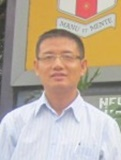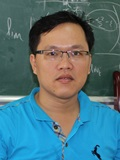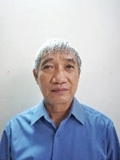Nhảy đến nội dung
x

## Applied Analysis Research Group

1. Introduction

Applied Analysis Research Group (AA-RG) was established in 2015 by Ton Duc Thang University (TDTU) with the objective of applying analytical and computational mathematics to solve real-world problems. AA-RG aims to develop and apply its results to applied analysis in many related fields of technologies such as Physics, Chemistry, Mechanics,… Its results will be published in prestigious international journals

2. Mission and vision

AA-RG is to enhance international publications of TDTU in prestigious journals indexed by ISI Web of Knowledge. Besides, this group is a part of the Faculty of Mathematics and Statistics of TDTU, which is to develop scientific research in terms of paper quality, training and researcher quantity.

3. Research topics

- Inverse Problems in Science and Engineering

- Numerical methods for Science and Engineering

- Partial Differential Equations

- Harmonic Analysis

- Numerical Analysis

- Variational Analysis

- Optimization

4. Current membersDr. Nguyen Huy Tuan Positions: • Head of Applied Analysis Research Group, Faculty of Mathematics and Statistics, Ton Duc Thang University, Ho Chi Minh City, Vietnam • Lecturer, Faculty of Mathematics and Statistics, Ton Duc Thang University, Ho Chi Minh City, Vietnam Areas of expertise: Differential equation, Numerical Analysis, PDEs, Nonparametric statisticsDr. Dao Nguyen Anh Positions: • Member of Applied Analysis Research Group, Faculty of Mathematics and Statistics, Ton Duc Thang University, Ho Chi Minh City, Vietnam • Lecturer, Faculty of Mathematics and Statistics, Ton Duc Thang University, Ho Chi Minh City, Vietnam Areas of expertise: Partial Differential EquationsDr. Luong Dang Ky Position: Member of Applied Analysis Research Group, Faculty of Mathematics and Statistics, Ho Chi Minh City, Ton Duc Thang University, Vietnam Areas of expertise: Harmonic Analysis, Real Analysis, Partial Differential EquationsDr. Ha Duy Hung Position: Member of Applied Analysis Research Group, Faculty of Mathematics and Statistics, Ton Duc Thang University, Ho Chi Minh City, Vietnam Areas of expertise: Harmonic Analysis, Integral transformsDr. Nguyen Bich Huy Position:  • Associate professor in Mathematics • Member of Applied Analysis Research Group, Faculty of Mathematics and Statistics, Ton Duc Thang University, Ho Chi Minh City, Vietnam Areas of expertise: Fixed Point Theory, Partial Differential EquationsDr. Nguyen Le Hoang Anh Position: Member of Applied Analysis Research Group, Faculty of Mathematics and Statistics, Ton Duc Thang University, Ho Chi Minh City, Vietnam Areas of expertise: Optimization, Variational AnalysisDr. Nguyen Huy Chieu Position: Member of Applied Analysis Research Group, Faculty of Mathematics and Statistics, Ton Duc Thang University, Ho Chi Minh City, Vietnam Areas of expertise: Optimization, Variational AnalysisDr. Duong Viet Thong Position: Member of Applied Analysis Research Group, Faculty of Mathematics and Statistics, Ton Duc Thang University, Ho Chi Minh City, Vietnam Areas of expertise: Fixed Point Theory, Iterative Methods, Equilibrium Problem, Variational Inequality Problem OptimizationDr. Dang Van Hieu Position: Member of Applied Analysis Research Group, Faculty of Mathematics and Statistics, Ton Duc Thang University, Ho Chi Minh City, Vietnam Areas of expertise: Numerical Methods, Numerical Analysis, Optimization, Variational Analysis and Equilibrium ProblemDr. Vo Hoang Hung Position: Member of Applied Analysis Research Group, Faculty of Mathematics and Statistics, Ton Duc Thang University, Ho Chi Minh City, Vietnam Areas of expertise: Mathematics Biology, Partial Differential EquationsNguyen Huu Can, MSc Position: Member of Applied Analysis Research Group, Faculty of Mathematics and Statistics, Ton Duc Thang University, Ho Chi Minh City, Vietnam Areas of expertise: Inverse Problems, Numerical Analysis, Partial Differential Equations

5. Former members (name, title, picture)Dr. Nguyen Hong Quan Position: Member of Applied Analysis Research Group, Faculty of Mathematics and Statistics, Ton Duc Thang University, Ho Chi Minh City, Vietnam

6. Publications (ISI or Scopus only)

34. Xuan Tran Dong, Tuan Nguyen Huy, Mokhtar Kirane (2018); Regularization and error estimate of infinite‐time ruin probabilities for Cramer‐Lundberg model; Mathematical Methods in the Applied Sciences (ISI).

33. Nguyen Huy Tuan, Vo Van Au, Nguyen Huu Can (2017); Regularization of initial inverse problem for strongly damped wave equation; Applicable Analysis (ISI).

32. Huy Tuan Nguyen, Mokhtar Kirane, Nam Danh Hua Quoc, Van Au Vo (2018); Approximation of an Inverse Initial Problem for a Biparabolic Equation; Mediterranean Journal of Mathematics (ISI).

31. Nguyen Huy Tuan, Mokhtar Kirane, Bandar Bin-Mohsin, Pham Thi Minh Tam (2017); Filter regularization for final value fractional diffusion problem with deterministic and random noise; Computers & Mathematics with Applications (ISI).

30. Erkan Nane, Nguyen HoangTuan, Nguyen Huy Tuan (2018); A random regularized approximate solution of the inverse problem for Burgers’ equation; Statistics & Probability Letters (ISI).

29. Mokhtar Kirane, Erkan Nane, Nguyen Huy Tuan (2017); On a backward problem for multidimensional Ginzburg–Landau equation with random data; Inverse Problems (ISI).

28. Nguyen Huy Tuan, Tran Dong Xuan, Nguyen Anh Triet, Daniel Lesnic (2018); On the Cauchy problem for a semilinear fractional elliptic equation; Applied Mathematics Letters (ISI).

27. Nguyen Huy Tuan, Tran Thanh Binh, Tran Quoc Viet, Daniel Lesnic (2015); On the Cauchy problem for semilinear elliptic equations; Journal of Inverse and Ill-posed Problems (ISI).

26. Nguyen Huy Tuan, Dinh Nguyen Duy Hai, Le Dinh Long, Van Thinh Nguyen, Mokhtar Kirane (2017); On a Riesz–Feller space fractional backward diffusion problem with a nonlinear source; Journal of Computational and Applied Mathematics (ISI).

25. Nguyen Huy Tuan, Le Duc Thang, Daniel Lesnic (2016); A new general filter regularization method for Cauchy problems for elliptic equations with a locally Lipschitz nonlinear source; Journal of Mathematical Analysis and Applications (ISI).

24. Nguyen Anh Dao, Quoc-Hung Nguyen (2017); Nonstationary Navier–Stokes equations with singular time-dependent external forces; Comptes Rendus Mathematique (ISI).

23. Nguyen Huy Tuan, Doan Vuong Nguyen, Vo Van Au, Daniel Lesnic (2017); Recovering the initial distribution for strongly damped wave equation; Applied Mathematics Letters (ISI).

22. Tuan Nguyen Huy, Mach Nguyet Minh, Mokhtar Kirane, Bandar Bin-Mohsin (2017); Regularization of an inverse nonlinear parabolic problem with time-dependent coefficient and locally Lipschitz source term; Journal of Mathematical Analysis and Applications (ISI).

21. Nguyen Huy Tuan, Le Dinh Long (2017); Fourier truncation method for an inverse source problem for space-time fractional diffusion equation; Electron. J. Differential Equations (ISI).

20. Nguyen Bich Huy, Bui The Quan, Nguyen Huu Khanh (2016); Existence and multiplicity results for generalized logistic equations; Nonlinear Analysis (ISI).

19. Hai Dinh Nguyen Duy, Tuan Nguyen Huy, Long Le Dinh, Gia Quoc Thong Le (2016); Inverse problem for nonlinear backward space-fractional diffusion equation; Journal of Inverse and Ill-posed Problems (ISI).

18. Nguyen Huy Tuan, Mokhtar Kirane, Vu Cam Hoan Luu, Bandar Bin-Mohsin (2016); A regularization method for time-fractional linear inverse diffusion problems; Electron. J. Differential Equations (ISI).

17. Nguyen Huy Tuan, Mokhtar Kirane, Luu Vu Cam Hoan, Le Dinh Long (2017); Identification and regularization for unknown source for a time-fractional diffusion equation; Computers & Mathematics with Applications (ISI).

16. Quoc Viet Tran, Mokhtar Kirane, Huy Tuan Nguyen, Van Thinh Nguyen (2017); Analysis and numerical simulation of the three-dimensional Cauchy problem for quasi-linear elliptic equations; Journal of Mathematical Analysis and Applications (ISI).

15. Nguyen Anh Dao, Jesus Ildefonso Díaz (2017); Existence and uniqueness of singular solutions of \$ p\$-Laplacian with absorption for Dirichlet boundary condition; Proceedings of the American Mathematical Society (ISI).

14. Dinh Thanh Duc, Ha Duy Hung, Luong Dang Ky (2016); On Weak∗∗-convergence in the Localized Hardy Spaces H1ρ(X)Hρ1(X) and its Application; Taiwanese Journal of Mathematics (ISI).

13. Ha Duy Hung, Duong Quoc Huy, Luong Dang Ky (2016); A note on weak∗∗-convergence in h1(Rd); Annals of Functional Analysis (ISI).

12. Nguyen Minh Chuong, Dao Van Duong, Ha Duy Hung (2016); Bounds for the Weighted Hardy-Cesàro Operator and its Commutator on Morrey-Herz Type Spaces; ZEITSCHRIFT FÜR ANALYSIS UND IHRE ANWENDUNGEN (ISI).

11. Nguyen Anh Dao (2016); Uniqueness of a very singular solution to nonlinear degenerate parabolic equations with absorption for Dirichlet boundary condition; Electron. J. Differential Equations (ISI).

10. Ha Duy Hung, Luong Dang Ky, Thai Thuan Quang; Norm of the Hausdorff Operator on the Real Hardy Space H1(R); Complex Analysis and Operator Theory (2017) (ISI).

9. Nguyen Huy Tuan, Vo Van Au, Vo Anh Khoa, Daniel Lesnic; Identification of the population density of a species model with nonlocal diffusion and nonlinear reaction; Inverse Problems, Vol. 33 (5) (2017), Article number 055019 (ISI).

8. Nguyen Anh Dao, Jesus Ildefonso Díaz; A gradient estimate to a degenerate parabolic equation with a singular absorption term: The global quenching phenomena; Journal of Mathematical Analysis and Applications, Vol. 437, pp. 445–473 (2016) (ISI).

7. Ha Duy Hung, Luong Dang Ky; New weighted multilinear operators and commutators of hardy-cesàro type; Acta Mathematica Scientia, Vol. 35, pp. 1411–1425 (2015) (ISI).

6. Ha Duy Hung, Luong Dang Ky; An hardy estimate for commutators of pseudo-differential operators; Taiwanese Journal of Mathematics, Vol. 19, pp. 1097-1109 (2015) (ISI).

5. Nguyen Huy Tuan, Vo Anh Khoa, Le Trong Lan, Tran The Hung; On the regularization of solution of an inverse ultraparabolic equation associated with perturbed final data; Journal of Inequalities and Applications, 2015:13 (2015) (ISI).

4. Nguyen Huy Tuan, Tran Thanh Binh, Nguyen Dang Minh, Truong Trong Nghia; An improved regularization method for initial inverse problem in 2-D heat equation; Applied Mathematical Modelling, Vol. 39, pp. 425–437 (2015) (ISI).

3. Nguyen Huy Tuan, Le Duc Thang, Vo Anh Khoa; A modified integral equation method of the nonlinear elliptic equation with globally and locally Lipschitz source; Applied Mathematics and Computation, Vol. 265, pp. 245–265 (2015) (ISI).

2. Vo Anh Khoa, Tuan Nguyen Huy, Le Trong Lan, Nguyen Thi Yen Ngoc; A finite difference scheme for nonlinear ultra-parabolic equations; Applied Mathematics Letters, Vol. 46, pp. 70–76 (2015) (ISI).

1. Van Thinh Nguyen, Huy Tuan Nguyen, Thanh Binh Tran and Anh Khoa Vo; On an inverse problem in the parabolic equation arising from groundwater pollution problem; Boundary Value problem, Boundary Value Problems, 2015:67 (2015) (ISI).